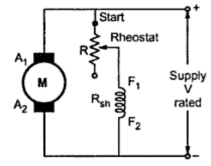### Speed Control of D.C. Shunt Motor : Flux Control

Speed Control of D.C. Shunt Motor (Part1)

1. Flux Control
As indicated by the speed equation, the speed is inversely proportional to the flux. The flux is dependent on the current through the shunt field winding. Thus flux can be controlled by adding a rheostat (variable resistance) in series with the shunt field winding, as shown in the Fig. 1.Fig. 1 Flux control of shunt motor
At the beginning the rheostat R is kept at minimum indicated as start in the Fig. 1. The supply voltage is at its rated value. So current through shunt field winding is also at its rated value. Hence the speed is also rated speed also called normal speed. Then the resistance R is increased due to which shunt field current Ish decreases, decreasing the flux produced. As N α  (1/Φ), the speed of the motor increases beyond its rated value.
Thus by this method, the speed control above rated value is possible. This is shown in the Fig. 2, by speed against field current curve. The curve shows the inverse relation between N and Φ as its nature is rectangular hyperbola.

It is mentioned that the rated values of electrical parameters should not be exceeded but the speed which is mechanical parameter can be increased upto twice its rated value.
1.1 Advantages of Flux Control Method
1. It provides relatively smooth and easy control.
2. Speed control above rated speed is possible.
3. As the field winding resistance is high, the field current is small. Hence power loss (R) in the external resistance is very small, which makes the method more economical and efficient.
4. As the field current is small, the size of rheostat required is small.
1.2 Disadvantages of Flux Control Method
1. The speed control below normal rated speed is not possible as flux can be increased only upto its rated value.
2. As flux reduces, speed increases. But high speed affects the commutation making motor operation unstable. So there is limit to the maximum speed above normal, possible by this method.